Home

# Rigidity times for a weakly mixing dynamical system which are not rigidity times for any irrational rotation

## Abstract

We construct an increasing sequence of natural numbers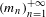$(m_{n})_{n=1}^{+\infty }$ with the property that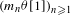$(m_{n}{\it\theta})_{n\geq 1}$ is dense in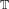$\mathbb{T}$ for any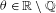${\it\theta}\in \mathbb{R}\setminus \mathbb{Q}$ , and a continuous measure on the circle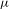${\it\mu}$ such that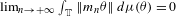$\lim _{n\rightarrow +\infty }\int _{\mathbb{T}}\Vert m_{n}{\it\theta}\Vert \,d{\it\mu}({\it\theta})=0$ . Moreover, for every fixed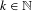$k\in \mathbb{N}$ , the set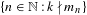$\{n\in \mathbb{N}:k\nmid m_{n}\}$ is infinite. This is a sufficient condition for the existence of a rigid, weakly mixing dynamical system whose rigidity time is not a rigidity time for any system with a discrete part in its spectrum.

Hide All

# Rigidity times for a weakly mixing dynamical system which are not rigidity times for any irrational rotation

## Metrics

### Full text viewsFull text views reflects the number of PDF downloads, PDFs sent to Google Drive, Dropbox and Kindle and HTML full text views.

Total number of HTML views: 0
Total number of PDF views: 0 *Loading metrics...

### Abstract viewsAbstract views reflect the number of visits to the article landing page.

Total abstract views: 0 *Loading metrics...

* Views captured on Cambridge Core between <date>. This data will be updated every 24 hours.

Usage data cannot currently be displayed